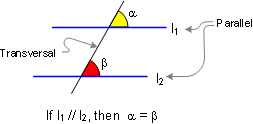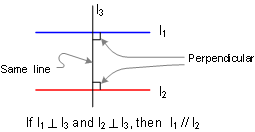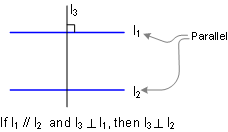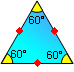Home > Proposed Problems > Previous, Key theorems, 1, 2, 3, 4, 5, 6, 7, 8, 9, 1011, 12, 13, 14, 15, 20, 30, 40, Next

# Geometry Help: Key Theorems or Facts You Should Know for the Proposed Problems

Do you know a theorem that could be useful?

Have you seen it before? Or have you seen the same problem in a slightly different form? Do you know a related problem?  Look at the unknown! And try to think of a familiar problem having the same or a similar unknown. George Polya, 1944.

Here is a summary of key theorems or facts you should know for the proposed problems.

Key Theorems:

 1. If two lines are parallel, each pair of corresponding angles are congruent. Also converse.2. If two lines are parallel, each pair of alternate interior angles are congruent. Also converse.3. If two lines are parallel, each pair of interior angles on the same side of the transversal are supplementary. Also converse.4. Two lines are parallel if they are perpendicular to the same line.5. If a line is perpendicular to one of two parallel lines, then it is also perpendicular to the other.6. The sum of the measures of the three angles of a triangle is 180.7. The measure of an exterior angle of a triangle equals the sum of the measures of the two non-adjacent interior angles.8. The sum of the measures of the acute angles of a right triangle is 90 (complementary).9. Triangle Congruence S.A.S. If two sides and the included angle of one triangle are congruent to the corresponding parts of another, then the triangles are congruent.10. Triangle Congruence A.S.A. If two angles and the included side of one triangle are congruent to the corresponding parts of another, then the triangles are congruent.11. Triangle Congruence S.S.S. If three sides of one triangle are congruent to the three sides of a second triangle, then the triangles are congruent.12. Right Triangle Congruence. Two right triangles are congruent if the hypotenuse and a leg of one triangle are congruent to the corresponding parts of the other triangle.13. An equilateral triangle is equiangular: 60-60-60. Also converse.14. Isosceles triangle: If two sides of a triangle are congruent, the angles opposite these sides are congruent. Also converse.15. Right triangle 45-45-90: the measure of either leg equals one-half the measure of the hypotenuse time.16. Right triangle 30-60-90: the measure of the side opposite the 30 angle is one-half the measure of the hypotenuse.Home  Email Last updated: December 2, 2007